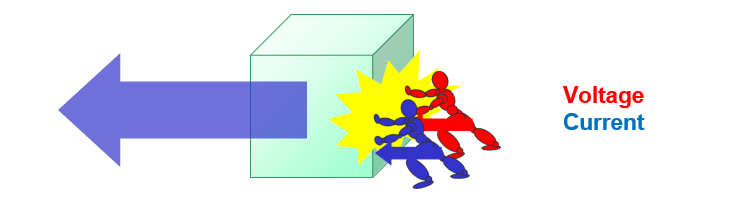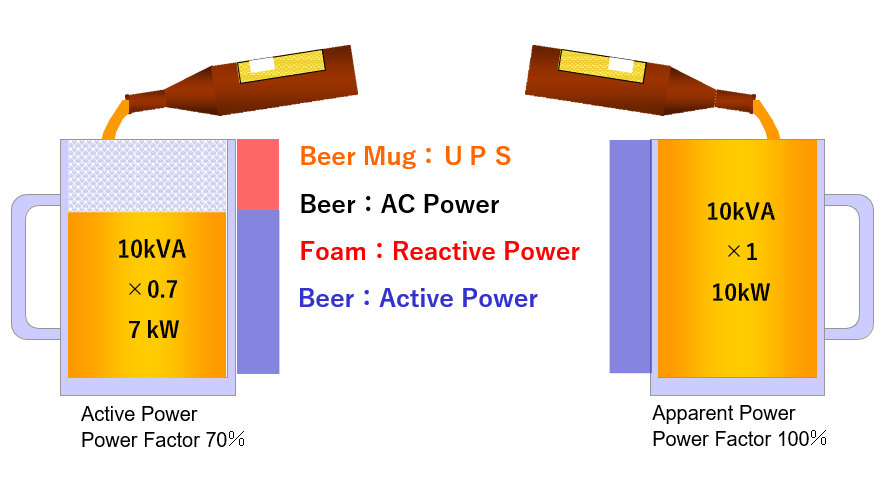# Vol.2: Active Power

## 1. What are Apparent Power and Active Power?

There are two types of capacity notation.

### Active power (kW): Power that actually does work

Since there is a deviation between the flow of voltage and current, the power that can theoretically be produced (apparent power) and the power that actually does work (active power) are indicated.

The deviation between voltage and current is called power factor.## 2. Power Factor

When the power factor is 100% (there is no deviation between voltage and current)When the power factor is 80% (there is a gap between voltage and current)The amount of work (active power) changes depending on the power factor.

## 3. Relation of capacity and power factor

Even with UPS of the same capacity, the active power varies depending on the power factor.